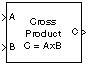# 3x3 Cross Product

Calculate cross product of two 3-by-1 vectors

• Library:
• Aerospace Blockset / Utilities / Math Operations

•## Description

The 3x3 Cross Product block computes cross (or vector) product of two vectors, A and B. The block generates a third vector, C, in a direction normal to the plane containing A and B, with magnitude equal to the product of the lengths of A and B multiplied by the sine of the angle between them. The direction of C follows the right-hand rule in turning from A to B. For related equations, see Algorithms.

## Ports

### Input

expand all

First cross product input, specified as a vector.

Example: `[10 2 3]`

Data Types: `double`

Second cross product input, specified as a vector.

Example: `[10 2 3]`

Data Types: `double`

### Output

expand all

Cross product, output as a vector.

Data Types: `double`

## Algorithms

The equations used to calculate A, B, and C are:

`$\begin{array}{c}A={a}_{1}i+{a}_{2}j+{a}_{3}k\\ B={b}_{1}i+{b}_{2}j+{b}_{3}k\\ C=A×B=|\begin{array}{ccc}i& j& k\\ {a}_{1}& {a}_{2}& {a}_{3}\\ {b}_{1}& {b}_{2}& {b}_{3}\end{array}|\\ =\left({a}_{2}{b}_{3}-{a}_{3}{b}_{2}\right)i+\left({a}_{3}{b}_{1}-{a}_{1}{b}_{3}\right)j+\left({a}_{1}{b}_{2}-{a}_{2}{b}_{1}\right)k\end{array}$`

## Extended Capabilities

### C/C++ Code GenerationGenerate C and C++ code using Simulink® Coder™.